# NCERT Solutions for Class 2 Maths Chapter 10

## NCERT Solutions for Class 2 Maths Chapter 10 - Add our Points

NCERT Class 2 Mathematics is an interesting book designed by experts for young minds. This book has beautiful illustrations to make kids understand the new concepts of Math and help them solve the problems. The syllabus is updated as per the CBSE norms. The 10th chapter of this book is ‘Add Our Points’. To clear the doubts, you can follow Class 2 Chapter 10 Solution prepared by the math experts of Vedantu. Every question in the exercise has been solved using the simplest method. Download NCERT Solutions for Class 2 Maths Chapter 10 Add Our Points in the PDF format to use it offline and study the chapter properly. You can use the solution as a reference and revise the chapter at your convenience.

Do you need help with your Homework? Are you preparing for Exams?
Study without Internet (Offline)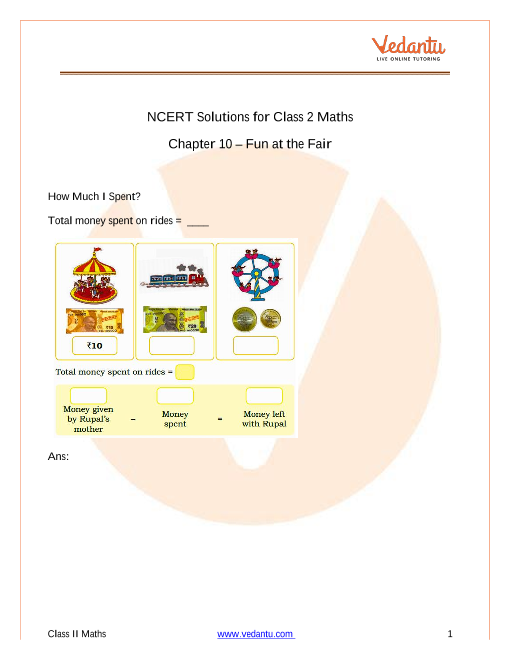## Access NCERT Solutions for class 2 Maths Chapter 10 – Add our points

1.

(a) Who won the game? ____________

(b) Who lost the game? ____________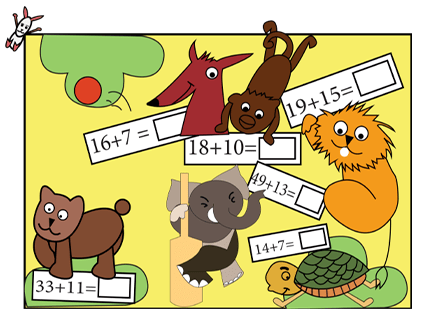Ans: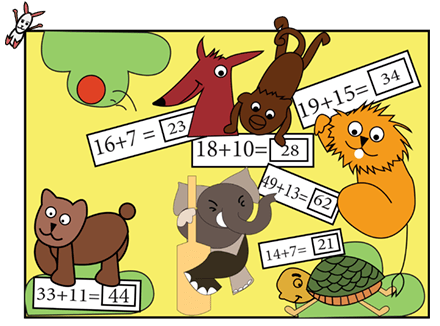(a). The Elephant has the highest number, so Elephants won.

(b). Tortoise has the lowest number of all, so tortoise lost the game.

2. Prabha is going to her grandmother, who lives in the next village. She wants to take $15\,kg$ sugarcane, $7\,kg$ apples and $8\,kg$ tomatoes. She has to cross a river. In the boat she can take $25\,kg$ weight. Can she carry all the things?

Ans: Add the total weight of all the things she wants to carry.

Total weight $=$  weight of sugarcane $+$weight of apples $+$ weight of tomatoes.

Total weight $= (15 + 7 + 8)\,kg$

Total weight $= 30\,kg$ .

No, Pratibha cannot carry all the vegetables in the boat because the total weight of the vegetables is $30\,kg$ but in the boat she can only take $25\,kg$ .

3.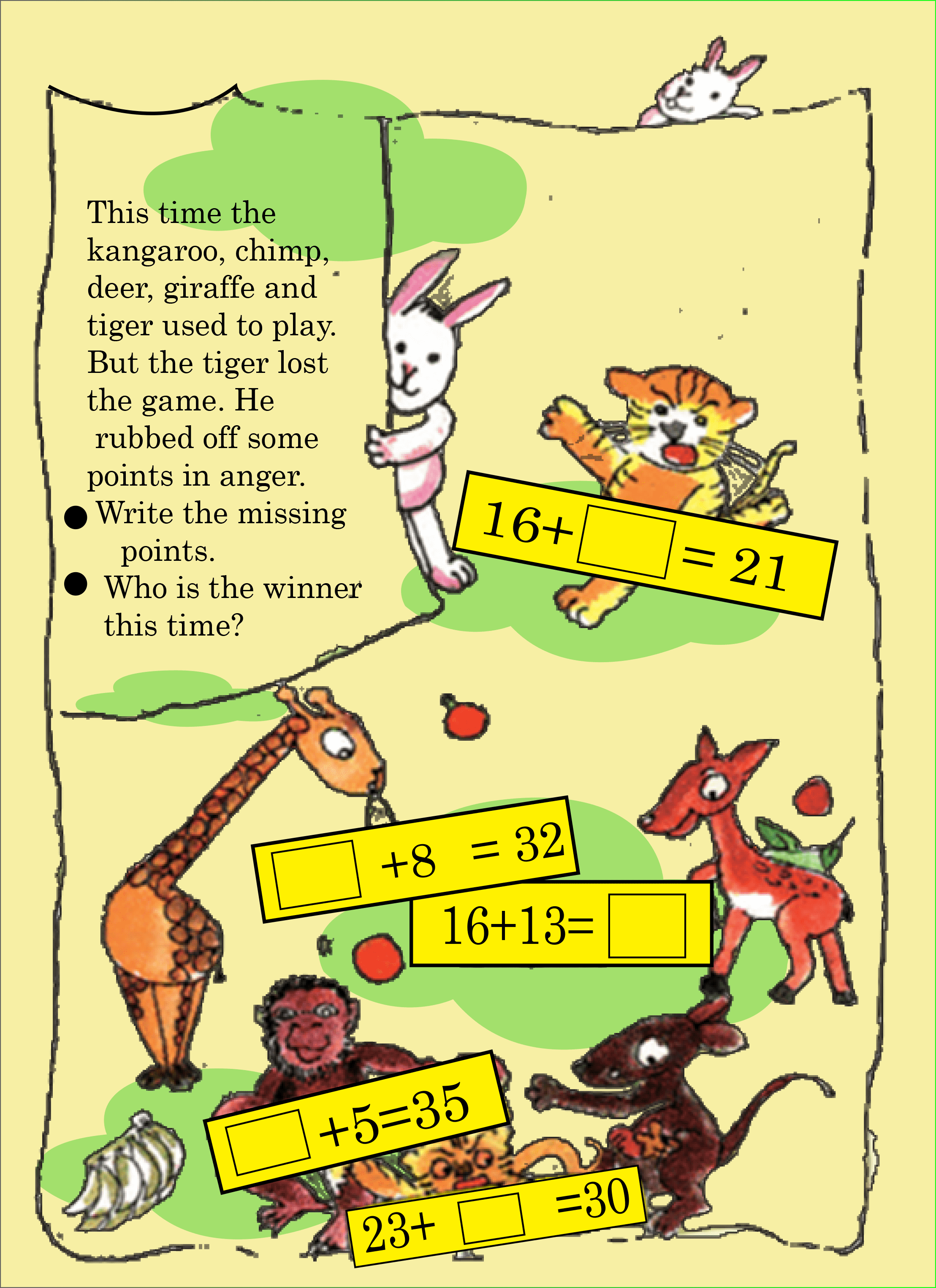Ans: This time, Monkey wins the game.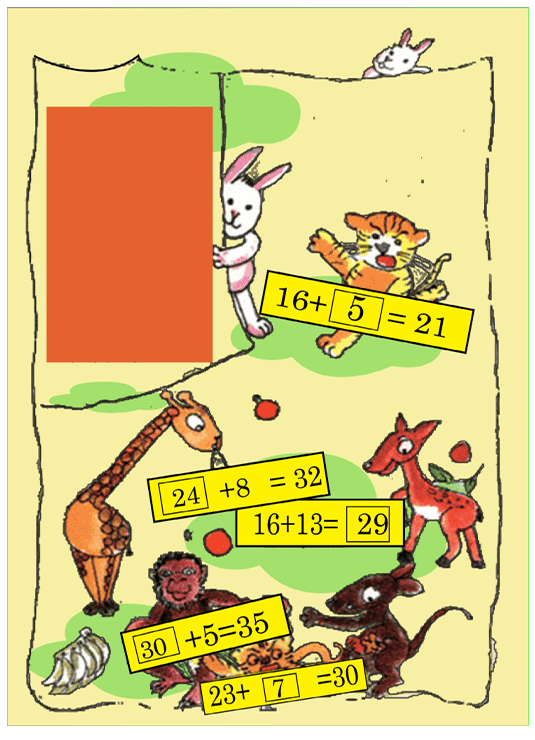4. Have you seen the two sides of a rupee coin?

Ans: Yes, I have seen the two sides of a rupee coin.

5. Which side has $1?$

Ans: In a coin, 1 is written on the side of the tail.

6. Chintu and Mintu went shopping. They bought some things. To pay they used notes and coins, but only two at a time.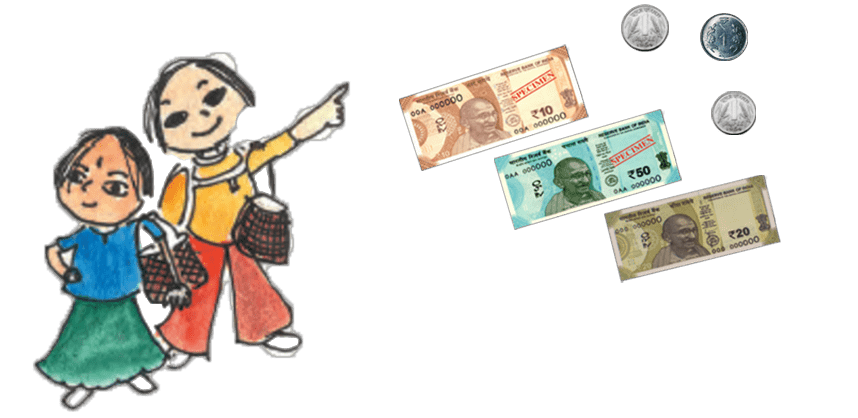Out of these, which two can they use to buy the things below? They can use the same note or coin more than one time.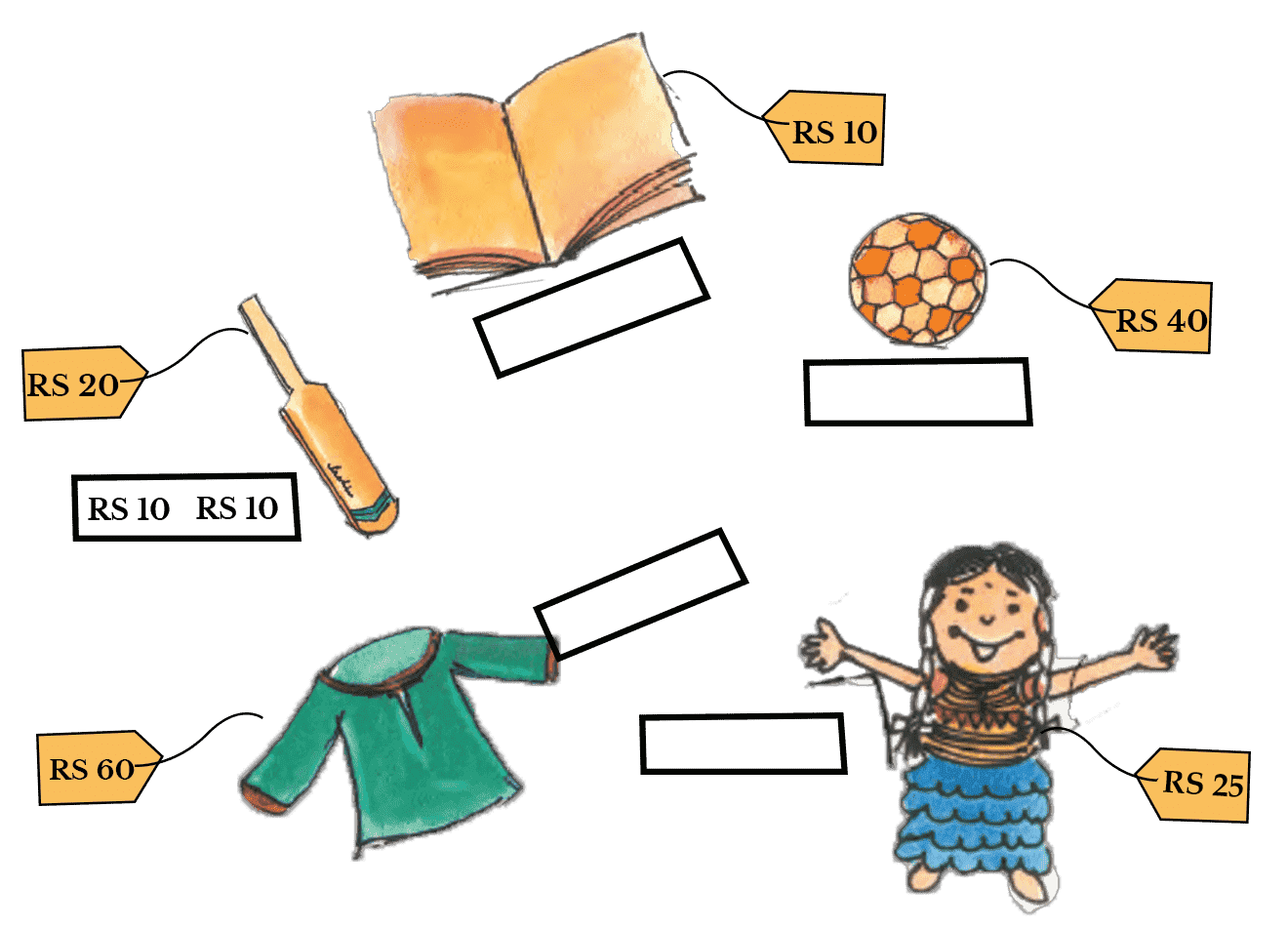Ans: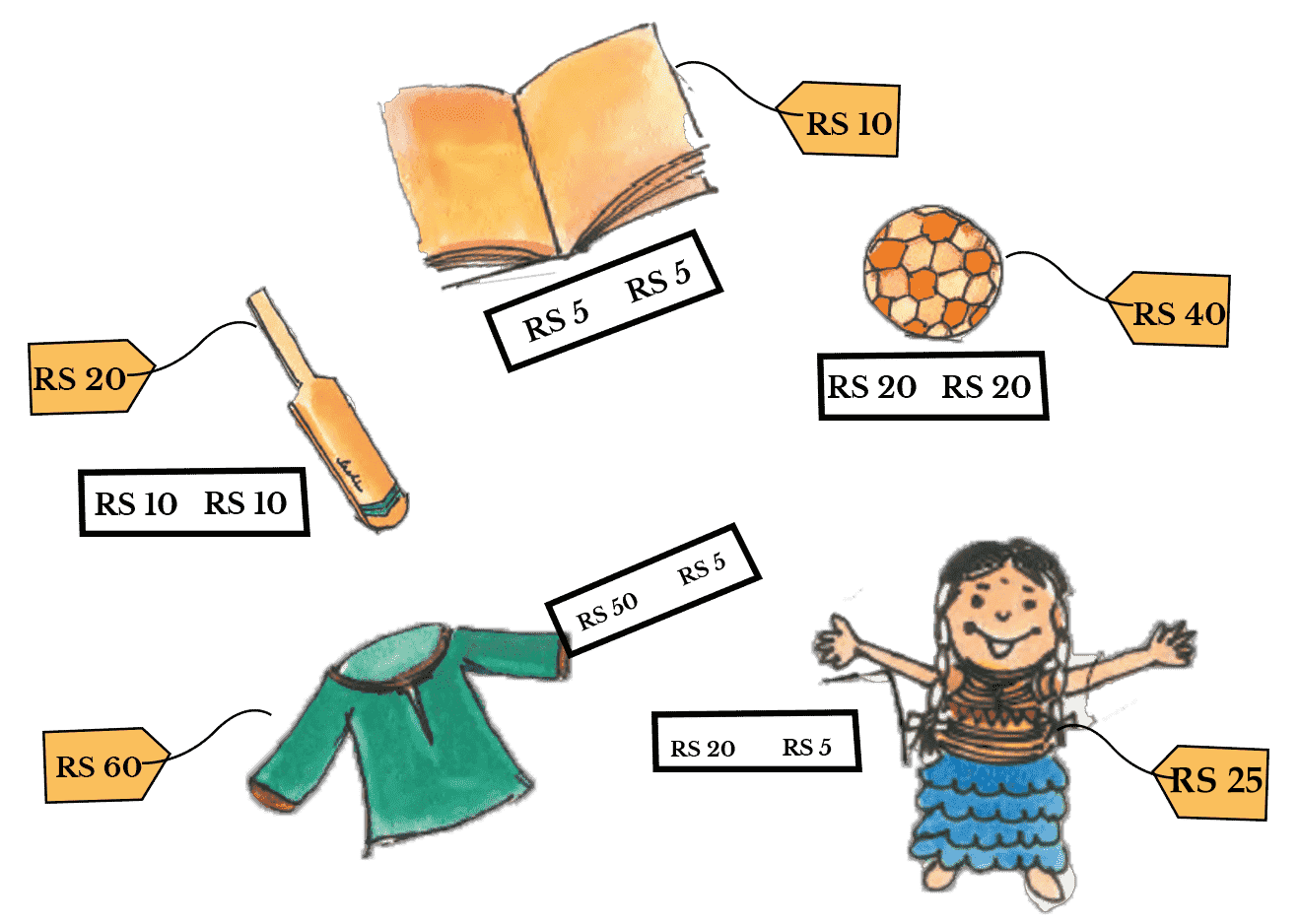7. Do these:

1. $5 + 5 + 7 = \_\_\_$

Ans: $5 + 5 + 7 = 17$

1. $6 + 5 + 4 = \_\_\_$

Ans: $6 + 5 + 4 = 15$

1. $9 + 4 + 1 = \_\_\_$

Ans: $9 + 4 + 1 = 14$

1. $7 + 3 + 8 = \_\_\_$

Ans: $7 + 3 + 8 = 18$

1. $8 + 3 + 2 = \_\_\_$

Ans: $8 + 3 + 2 = 13$

8. A lizard moves from one hole to another. As it moves, it eats insects hidden in the hole. The number of insects in each hole is shown. The lizard can move only along the lines.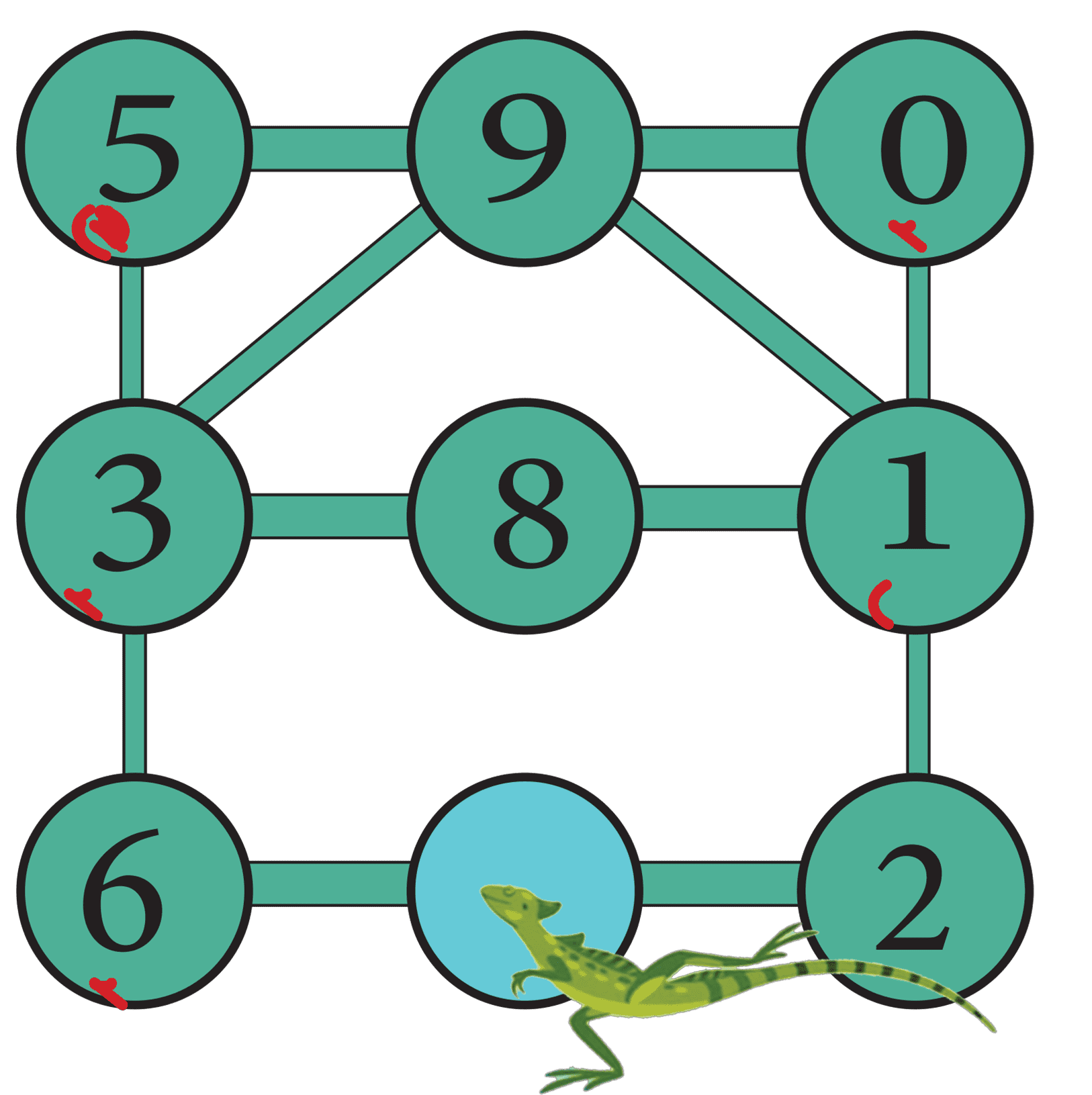Starting from the blue hole in the picture, the lizard goes to three holes to eat  $18$ insects. This is the path the lizard takes.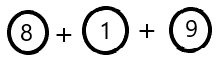$= 18$

(a) What path can the lizard take to eat $12$ insects ?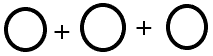$= 12$

Ans: The path in which the lizard can take  $12$ insects is: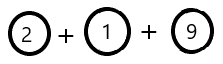(b) What path can the lizard take to eat $20$ insects?$= 20$

Ans: The path in which the lizard can take  $20$ insects is: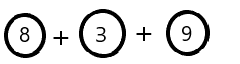(c) This time the lizard goes to four holes to eat insects. What path does the lizard take to eat $18$ insects?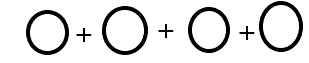$= 18$

Ans: The path in which the lizard can take  $18$ insects is: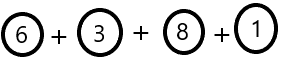(d) What path does the lizard take for $12$ insects?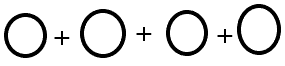$= 12$

Ans: The path in which the lizard can take  $12$ insects is: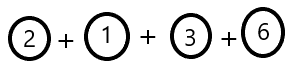## Add Our Points: Class 2th Maths Chapter 10

How can a mathematics chapter be made interesting for kids? Ch 10 Class 2 Maths by Vedantu is the best example that follows such methods. This chapter is designed to deliver the basic concepts of addition. Maths Class 2 Chapter 10 has a beautiful set of illustrations with funny pictures of animals helping kids understand the concept of addition. This method of concept delivery is unique. Students will also find it fascinating to study. Young minds will find it quite engaging as this chapter has beautiful pictures. Every problem is represented using pictures of animals asking conceptual questions to kids. This will make kids study on their own as the format of the chapter is quite motivating.

If you face any doubt while preparing the chapter, you can use NCERT Solution for Class 2 Maths Chapter 10 prepared by experts to find the answers. All the answers to the questions in the exercise are framed in such a way that every student can understand the concepts well and use them to solve problems conveniently in an exam. The chapter is quite engaging. Children will be happy to complete the chapter due to its fun illustrations. Using the solution will make it even easier for students to prepare the chapter well and score better.

This chapter is important for children to develop the concepts of addition. You can consider it as the foundation of students on which the further chapters will rely on. Hence, studying this chapter will be essential. To ensure a proper understanding of the chapter, you can use Class 2 Maths Ch 10 NCERT Solutions and learn how to approach the problems in the exercise. It will help children to develop the concept on their own and solve the problems in an exam easily.

### Why Should You Use CBSE Class 2 Maths Chapter 10 Solutions?

The prime reason for using the CBSE Class 2 Maths Chapter 10 solutions is to create a strong base of addition. Developing the concept of addition is very important in Class 2 students. The rest of the chapters in the book will depend on this development. The students will also find more confidence in studying this chapter when a solution is there with the study material. The answers in the solution are given in an easy format with proper illustration so that every student can grab the concepts well and solve the problems on their own.

Add Our Points Class 2 NCERT Solutions are ideal to use during practising and revising the chapter. You can rest assured that the conceptual questions are answered following the level of the kids. They are just being introduced to the concept of addition. This is where the solution becomes extremely important for their learning. This solution will also make the study schedule more convenient and time-efficient. Students will be able to reduce their preparation time to a huge extent. The solution is available in PDF format. Add more convenience and confidence in preparing this chapter and score well in the exams.

## FAQs on NCERT Solutions for Class 2 Maths Chapter 10

1. What is the Prime Benefit of Using NCERT Solutions Class 2 Maths Add Our Points?

Students of Class 2 will be introduced to the concept of addition. Apart from the illustration of the chapter, students will be able to understand the concept by following the solution provided by Vedantu. It is an important step to take. Adding a solution to the study material will be highly beneficial for the kids.

2. How Should You Prepare Class 2 Maths Chapter 10 Add Our Points?

Students should follow how the teachers are explaining the concept of this chapter in the classroom. They can also take help from the teachers of Vedantu online. Once the chapter is complete, they can proceed to the exercise and try solving the problems on their own. They can refer to NCERT Solutions Class 2 Maths Chapter 10 to gain more confidence.

3. Are the methods taught in Class 2 Chapter 10 Maths easy?

The methods used to solve the problems of all the exercises are provided in the simplest way. They are easy as long as the students refer to the Vedantu guide that is found on their official website (vedantu.com). The Vedantu solutions that are provided are in a step-by-step manner. These solutions are verified by the subject matter experts and are provided in the most interactive way. They also follow the guidelines set forth by the CBSE Board.

4. How is this chapter interesting or fascinating to study?

The Vedantu guide provides pictures of the animals trying to explain the concept by posing questions to the students. This interactive way of explanation is proven to be interesting and fascinating. The solutions at Vedantu are provided by subject matter experts in a simple and step-by-step manner. These solutions are provided by following the guidelines of NCERT and CBSE Board and also by the level of understanding of the children.

5. What is this chapter mainly built upon?

This chapter mainly focuses on and aims at the basic addition of numbers. The concept of addition serves as the foundation for almost all the chapters in the later chapters and classes. Vedantu offers solutions to all the exercises of this chapter in a most simplified and step-by-step manner. The solutions are prepared in an interactive way for the students to understand in a better way. The NCERT solutions for Class 2 can also be downloaded free of cost to study offline as well.

6. Does this chapter find applications in real life?

Adding numbers is a basic concept that every student must know. It forms the foundation for higher grade mathematics. Once a strong base is created, developing the rest of the chapters becomes easy. It finds application in adding the amount of money, the number of pencils, pens, etc. It helps in exercising the brains of the students.  The Vedantu Guide offers the complete student guide for the children that includes revision notes, important questions, mock tests, solutions to all the exercises in a simplified way, and doubt clearing sessions.

7. What are the different methods used while adding numbers?

Different ways that are opted for learning addition are pictorial representation, addition charts, using physical objects, using a number line, etc. In addition to forming the base for all the upcoming chapters, it is very important for the students to learn these concepts. The material provided on Vedantu's official website and the app focuses on making the foundation of addition stronger for the students to apply the concepts in real life.SHARETWEETSHARESUBSCRIBE